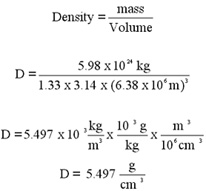Density

Density is a derived quantity that has proven to be very useful in understanding the behavior of matter around us. We define mass density to be the quotient of mass divided by volume. Common units for density are grams/cm^3 or kg/m^3. A standard reference point for density is the density of water which is 1 gram/cm^3 or 1000 kg/m^3. A box that has dimensions of 10 cm x 10 cm x 10 cm has volume of 1000 cm^3 and therefore holds 1000 grams or 1 kg of water. (You should know that the density of water varies slightly with temperature. Water is at its densest at +4 oC.)
Consult an older text and see the term specific gravity defined to be the density of a substance divided by the density of water. This term has no units

Legend has it that Archimedes used the concept of density to prove that the crown commissioned by King Hiero II of Syracuse was fashioned with baser elements. This is the "eureka" story. There is some question as to the veracity of that tale. See the argument at http://www.mcs.drexel.edu/~crorres/Archimedes/Crown/CrownIntro.html

The density of each element on the periodic table, including gold, is known. See a listing at
http://web.mit.edu/3.091/www/pt/pert6.htmlThe least dense element is hydrogen; the densest elements are found among the precious metals of period 6. It is curious to speculate how these elements are distributed within the planet. The densities of materials where we live (on the outer crust of the Earth) are relatively small. Water of course in 1 g/cm^3. The density of things living or recently alive is very close to 1 g/cm^3. When we think of heavy stuff, materials such a granite come to mind; granite is a mixture of elements having a range of density in the neighborhood of 2.5 - 2.8 g/cm^3.

The average density of the Earth is calculated at left and comes in at a value twice the density of granite. This would imply that the bulk of the Earth is composed of heavier elements, probably metals, at the core of the planet. (So much for "third 'rock' from the sun".) A molten metal core swirling at the center of a rotating planet also provides a plausible explanation for the cause of Earth's magnetic field.

It is said that an object that floats in water (or in any other liquid for that matter) will displace its own weight. Let's assume that we have a block having a mass of 500 g and a volume of 1000 cm^3. This yields a density of .5 g/cm^3. That means that the block floats, sinking into the water until it has displaced its weight of 500 g of water. That in turn, means that the object floats only when it has displaced 500 cm^3 of water. 500 g of block displacing 500 cm^3 of water means we can say that an object will float only when its effective density is 1.00.